## How to calculate quarter and year from date in Excel?

If you have a column of date, now, you want to convert the date to quarter and year only to get the following result. Are there any quick ways to solve it in Excel?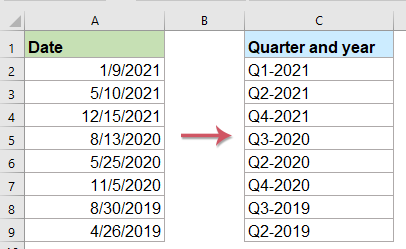#### Convert date to quarter and year with formula

Here is a simple formula can help you to calculate the quarter and year from the given date, please do as follows:

Enter the following formula into a blank cell where you want to output the calculate result, and then drag the fill handle down to the cells to apply this formula, and the date has been displayed in quarter and year format, see screenshot:

="Q" &INT((MONTH(A2)+2)/3) & "-" & YEAR(A2)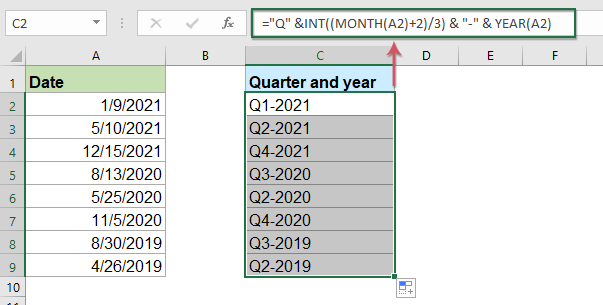#### Convert date to quarter only with formula

If you just want to get the quarter from the given date, please apply the below formula:

="Q"&ROUNDUP(MONTH(A2)/3,0)

Then, drag the fill handle down to the cells for filling this formula, only the quarter is displayed based on the date, see screenshot: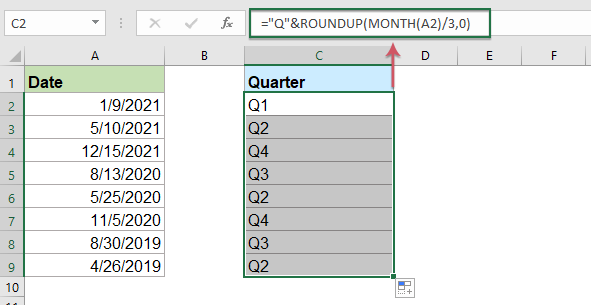#### Convert date to quarter and year with a handy feature

If you have Kutools for Excel, with its Convert date to quarter feature, you can get the quarter and year format from te specific dates without remembering any formulas.

Note:To apply these Convert date to quarter features, firstly, you should download the Kutools for Excel, and then apply the features quickly and easily.

After installing Kutools for Excel, please do as follows:

1. Click to select a cell where you want to output the result, see screenshot: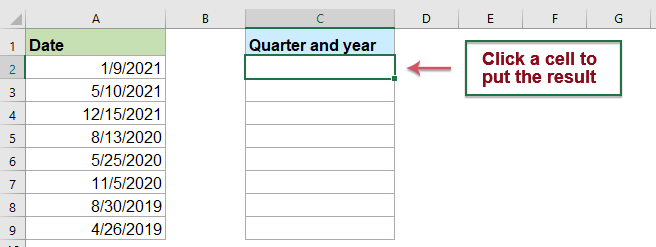2. And then click Kutools > Formula Helper > Formula Helper, see screenshot: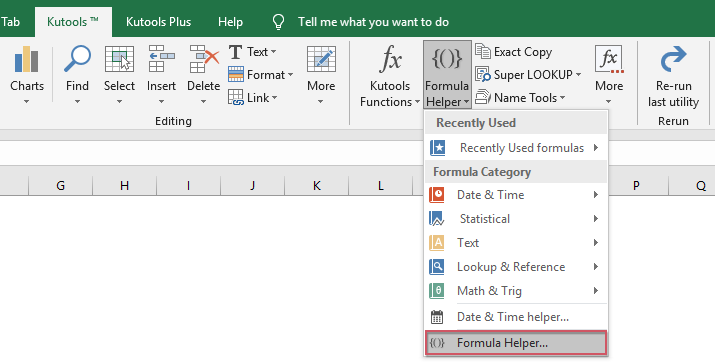3. In the Formulas Helper dialog box, please do the following operations:

• Select Date from the Formula Type drop down list;
• In the Choose a formula list box, click to select Convert date to quarter option;
• Then, in the Arguments input section, select the cell containing the date that you want to convert in the Date textbox.
Tips: In the Date textbox, you should change the default absolute cell reference to relative cell reference for dragging the formula correctly.4. And then, click Ok button, the first result will be calculated, then drag the fill handle for fill the formula to other cells, see screenshot: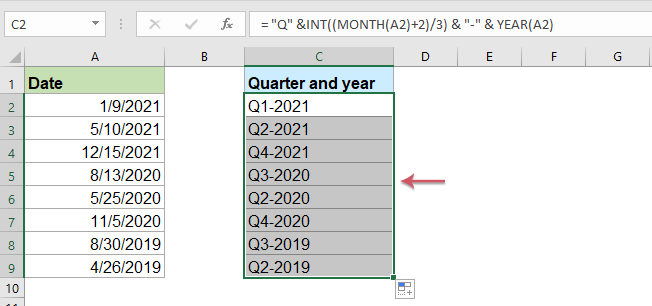#### Convert quarter and year to date with formula

Sometimes, you may want to convert the quarter and year format cells to the normal date which is the first day of quarter as below screenshot shown: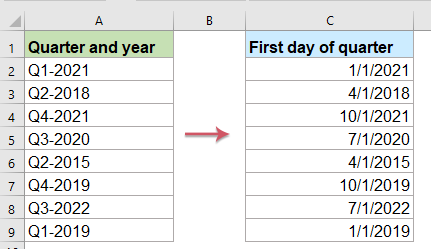For getting the first day of quarter from the quarter and year cell, please apply the below formula:

=DATE(RIGHT(A2,4),(MID(A2,2,1)*3)-2,1)

Then, drag the fill handle down to the cells for filling this formula, and all the fist days of quarter have been extracted at once, see screenshot: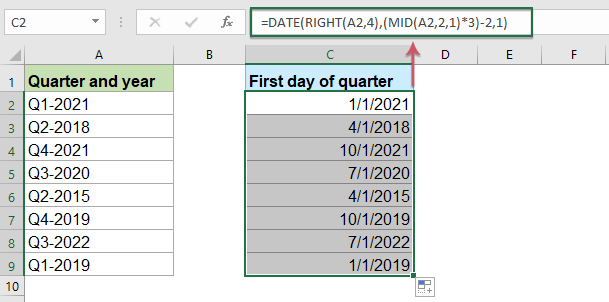#### More relative articles:

• Extract Month And Year Only From Date In Excel
• If you have a list of date format, now, you want to extract only the month and year from date as left screenshot shown, how could you extract month and year from the date quickly and easily in Excel?
• Sum Values Based On Month And Year In Excel
• If you have a range of data, column A contains some dates and column B has the number of orders, now, you need to sum the numbers based on month and year from another column. In this case, I want to calculate the total orders of January 2016 to get the following result. And this article, I will talk about some tricks to solve this job in Excel.
• Calculate Number Of Days In A Month Or A Year In Excel
• As we all know, there are leap years and common years where leap year has 366 days and common year has 365 days. To calculate the number of days in a month or a year based on a date as below screenshot shown, this article will help you.

### Best Office Productivity Tools

 Popular Features: Find, Highlight or Identify Duplicates   |  Delete Blank Rows   |  Combine Columns or Cells without Losing Data   |   Round without Formula ... Super Lookup: Multiple Criteria VLookup  |   Multiple Value VLookup  |   VLookup Across Multiple Sheets   |   Fuzzy Lookup .... Advanced Drop-down List: Quickly Create Drop Down List   |  Dependent Drop Down List   |  Multi-select Drop Down List .... Column Manager: Add a Specific Number of Columns  |  Move Columns  |  Toggle Visibility Status of Hidden Columns  |  Compare Ranges & Columns ... Featured Features: Grid Focus   |  Design View   |   Big Formula Bar   |  Workbook & Sheet Manager   |  Resource Library (Auto Text)   |  Date Picker   |  Combine Worksheets   |  Encrypt/Decrypt Cells   |  Send Emails by List   |  Super Filter   |   Special Filter (filter bold/italic/strikethrough...) ... Top 15 Toolsets:  12 Text Tools (Add Text, Remove Characters, ...)   |   50+ Chart Types (Gantt Chart, ...)   |   40+ Practical Formulas (Calculate age based on birthday, ...)   |   19 Insertion Tools (Insert QR Code, Insert Picture from Path, ...)   |   12 Conversion Tools (Numbers to Words, Currency Conversion, ...)   |   7 Merge & Split Tools (Advanced Combine Rows, Split Cells, ...)   |   ... and more

Supercharge Your Excel Skills with Kutools for Excel, and Experience Efficiency Like Never Before. Kutools for Excel Offers Over 300 Advanced Features to Boost Productivity and Save Time.  Click Here to Get The Feature You Need The Most...#### Office Tab Brings Tabbed interface to Office, and Make Your Work Much Easier

• Enable tabbed editing and reading in Word, Excel, PowerPoint, Publisher, Access, Visio and Project.
• Open and create multiple documents in new tabs of the same window, rather than in new windows.
• Increases your productivity by 50%, and reduces hundreds of mouse clicks for you every day!Rated 5 out of 5 · 1 ratings
This comment was minimized by the moderator on the site
In case you want to find the Financial QTR in a Macro, Assuming that Date is on the LEFT COLUMN.

ActiveCell.FormulaR1C1 ="=IF(AND(MONTH(RC[-1])>=1,MONTH(RC[-1])<=3),"Q4",IF(AND(MONTH(RC[-1])>=4,MONTH(RC[-1])<=6),"Q1",IF(AND(MONTH(RC[-1])>=7,MONTH(RC[-1])<=9),"Q2","Q3")))"
This comment was minimized by the moderator on the site
This formula works but I don't understand why. Can someone please explain? Thanks

="Q"&ROUNDUP(MONTH(B3)/3,0)
This comment was minimized by the moderator on the site

Rated 5 out of 5
This comment was minimized by the moderator on the site
Hello, Dort,
The explanation of this formula is:
MONTH(B3)：This MONTH function extracts the month number from the date cell;
MONTH(B3)/3: Divide by 3, the number 3 represents the months per quarter;
ROUNDUP(MONTH(B3)/3,0): This ROUNDUP function is used to round up the value to the nearest whole number;
"Q"&ROUNDUP(MONTH(B3)/3,0): If you would like to write Quarter or Q in front of each number, you can concatenate the numeric result from ROUNDUP with the text “Quarter “ or "Q" by using the & character.

Thank you!
This comment was minimized by the moderator on the site